SNAP Library 6.0, Developer Reference  2020-12-09 16:24:20 SNAP, a general purpose, high performance system for analysis and manipulation of large networks
TBPGraph Class Reference

Bipartite graph. More...

`#include <graph.h>`

Collaboration diagram for TBPGraph: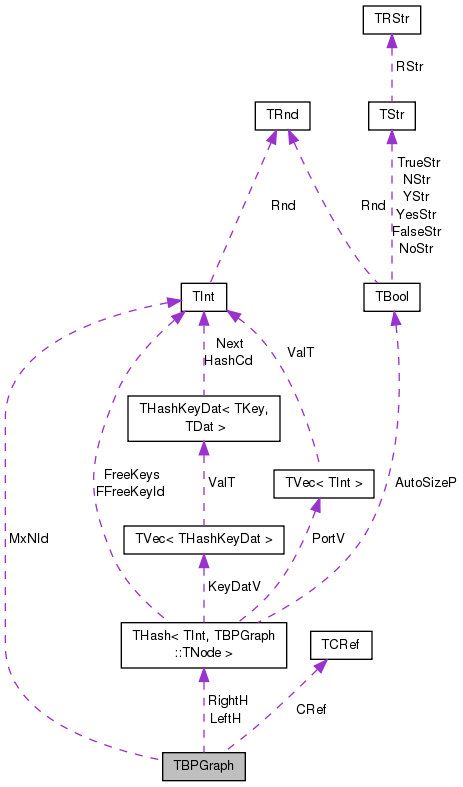[legend]

## Classes

class  TEdgeI
Edge iterator. Only forward iteration (operator++) is supported. More...

class  TNode

class  TNodeI
Node iterator. Only forward iteration (operator++) is supported. More...

## Public Types

enum  TNodeTy { bgsUndef, bgsLeft, bgsRight, bgsBoth }

typedef TBPGraph TNet

typedef TPt< TBPGraphPNet

## Public Member Functions

TBPGraph ()

TBPGraph (const int &Nodes, const int &Edges)
Constructor that reserves enough memory for a graph of Nodes (LeftNodes+RightNodes) nodes and Edges edges. More...

TBPGraph (const TBPGraph &BPGraph)
!! Reserve(Nodes, Edges); } More...

TBPGraph (TSIn &SIn)

void Save (TSOut &SOut) const
Saves the graph to a (binary) stream SOut. More...

bool HasFlag (const TGraphFlag &Flag) const
Allows for run-time checking the type of the graph (see the TGraphFlag for flags). More...

TBPGraphoperator= (const TBPGraph &BPGraph)

int GetNodes () const
Returns the total number of nodes in the graph. More...

int GetLNodes () const
Returns the number of nodes on the 'left' side of the biparite graph. More...

int GetRNodes () const
Returns the number of nodes on the 'right' side of the biparite graph. More...

int AddNode (int NId=-1, const bool &LeftNode=true)
Adds a node of ID NId to the graph. More...

Adds a node of ID NodeI.GetId() to the graph. More...

void DelNode (const int &NId)
Deletes node of ID NId from the graph. More...

void DelNode (const TNode &NodeI)
Deletes node of ID NodeI.GetId() from the graph. More...

bool IsNode (const int &NId) const
Tests whether ID NId is a node. More...

bool IsLNode (const int &NId) const
Tests whether ID NId is a 'left' side node. More...

bool IsRNode (const int &NId) const
Tests whether ID NId is a 'right' side node. More...

int GetMxNId () const
Returns an ID that is larger than any node ID in the graph. More...

TNodeI BegNI () const
Returns an iterator referring to the first node in the graph. More...

TNodeI EndNI () const
Returns an iterator referring to the past-the-end node in the graph. More...

TNodeI GetNI (const int &NId) const
Returns an iterator referring to the node of ID NId in the graph. More...

TNodeI BegLNI () const
Returns an iterator referring to the first 'left' node in the graph. More...

TNodeI EndLNI () const
Returns an iterator referring to the past-the-end 'left' node in the graph. More...

TNodeI BegRNI () const
Returns an iterator referring to the first 'right' node in the graph. More...

TNodeI EndRNI () const
Returns an iterator referring to the past-the-end 'right' node in the graph. More...

int GetEdges () const
Returns the number of edges in the graph. More...

int AddEdge (const int &LeftNId, const int &RightNId)
Adds an edge between a node LeftNId on the left and a node RightNId on the right side of the bipartite graph. More...

Adds an edge between EdgeI.GetLNId() and EdgeI.GetRNId() to the graph. More...

void DelEdge (const int &LeftNId, const int &RightNId)
Deletes an edge between a node LeftNId on the left and a node RightNId on the right side of the bipartite graph. More...

bool IsEdge (const int &LeftNId, const int &RightNId) const
Tests whether an edge between node IDs LeftNId and RightNId exists in the graph. More...

TEdgeI BegEI () const
Returns an iterator referring to the first edge in the graph. More...

TEdgeI EndEI () const
Returns an iterator referring to the past-the-end edge in the graph. More...

TEdgeI GetEI (const int &EId) const
Not supported/implemented! More...

TEdgeI GetEI (const int &LeftNId, const int &RightNId) const
Returns an iterator referring to edge (LeftNId, RightNId) in the graph. More...

int GetRndNId (TRnd &Rnd=TInt::Rnd)
Returns an ID of a random node in the graph. More...

int GetRndLNId (TRnd &Rnd=TInt::Rnd)
Returns an ID of a random 'left' node in the graph. More...

int GetRndRNId (TRnd &Rnd=TInt::Rnd)
Returns an ID of a random 'right' node in the graph. More...

TNodeI GetRndNI (TRnd &Rnd=TInt::Rnd)
Returns an interator referring to a random node in the graph. More...

void GetNIdV (TIntV &NIdV) const
Gets a vector IDs of all nodes in the bipartite graph. More...

void GetLNIdV (TIntV &NIdV) const
Gets a vector IDs of all 'left' nodes in the bipartite graph. More...

void GetRNIdV (TIntV &NIdV) const
Gets a vector IDs of all 'right' nodes in the bipartite graph. More...

bool Empty () const
Tests whether the bipartite graph is empty (has zero nodes). More...

void Clr ()
Deletes all nodes and edges from the bipartite graph. More...

void Reserve (const int &Nodes, const int &Edges)
Reserves memory for a biparite graph of Nodes nodes and Edges edges. More...

Defragments the biparite graph. More...

bool IsOk (const bool &ThrowExcept=true) const
Checks the bipartite graph data structure for internal consistency. More...

void Dump (FILE *OutF=stdout) const
Print the biparite graph in a human readable form to an output stream OutF. More...

## Static Public Member Functions

static PBPGraph New ()
Static constructor that returns a pointer to the graph. Call: PBPGraph BPGraph = TBPGraph::New();. More...

static PBPGraph New (const int &Nodes, const int &Edges)
Static constructor that returns a pointer to the graph and reserves enough memory for Nodes nodes and Edges edges. More...

Static constructor that loads the graph from a stream SIn and returns a pointer to it. More...

static PBPGraph GetSmallGraph ()
Returns a small graph on 2 'left', 3 'right' nodes and 4 edges. More...

## Private Attributes

TCRef CRef

TInt MxNId

THash< TInt, TNodeLeftH

THash< TInt, TNodeRightH

## Friends

class TPt< TBPGraph >

## Detailed Description

Bipartite graph.

Definition at line 936 of file graph.h.

## Member Typedef Documentation

 typedef TPt TBPGraph::PNet

Definition at line 939 of file graph.h.

 typedef TBPGraph TBPGraph::TNet

Definition at line 938 of file graph.h.

## Member Enumeration Documentation

 enum TBPGraph::TNodeTy
Enumerator
bgsUndef
bgsLeft
bgsRight
bgsBoth

Definition at line 940 of file graph.h.

940 { bgsUndef, bgsLeft, bgsRight, bgsBoth } TNodeTy; // left or right hand side node
TNodeTy
Definition: graph.h:940

## Constructor & Destructor Documentation

 TBPGraph::TBPGraph ( )
inline

Definition at line 1053 of file graph.h.

1053 : CRef(), MxNId(0), LeftH(), RightH() { }
TCRef CRef
Definition: graph.h:1045
TInt MxNId
Definition: graph.h:1046
THash< TInt, TNode > RightH
Definition: graph.h:1048
THash< TInt, TNode > LeftH
Definition: graph.h:1047

Here is the caller graph for this function:TBPGraph::TBPGraph ( const int & Nodes, const int & Edges )
inlineexplicit

Constructor that reserves enough memory for a graph of Nodes (LeftNodes+RightNodes) nodes and Edges edges.

Definition at line 1055 of file graph.h.

1055 : MxNId(0) { }
TInt MxNId
Definition: graph.h:1046
 TBPGraph::TBPGraph ( const TBPGraph & BPGraph )
inline

!! Reserve(Nodes, Edges); }

Definition at line 1056 of file graph.h.

1056 : MxNId(BPGraph.MxNId), LeftH(BPGraph.LeftH), RightH(BPGraph.RightH) { }
TInt MxNId
Definition: graph.h:1046
THash< TInt, TNode > RightH
Definition: graph.h:1048
THash< TInt, TNode > LeftH
Definition: graph.h:1047
 TBPGraph::TBPGraph ( TSIn & SIn )
inline

Definition at line 1058 of file graph.h.

1058 : MxNId(SIn), LeftH(SIn), RightH(SIn) { }
TInt MxNId
Definition: graph.h:1046
THash< TInt, TNode > RightH
Definition: graph.h:1048
THash< TInt, TNode > LeftH
Definition: graph.h:1047

## Member Function Documentation

 int TBPGraph::AddEdge ( const int & LeftNId, const int & RightNId )

Adds an edge between a node LeftNId on the left and a node RightNId on the right side of the bipartite graph.

Definition at line 705 of file graph.cpp.

References TStr::Fmt(), and IAssertR.

Referenced by TSnap::GenRewire().

705  {
706  const bool IsLL = IsLNode(LeftNId), IsLR = IsRNode(LeftNId);
707  const bool IsRL = IsLNode(RightNId), IsRR = IsRNode(RightNId);
708  IAssertR((IsLL||IsLR)&&(IsRL||IsRR), TStr::Fmt("%d or %d is not a node.", LeftNId, RightNId).CStr());
709  IAssertR(LeftNId!=RightNId, "No self-edges are allowed.");
710  IAssertR((IsLL&&!IsLR&&!IsRL&&IsRR)||(!IsLL&&IsLR&&IsRL&&!IsRR), "One node should be on the 'left' and the other on the 'right'.");
711  const int LNId = IsLL ? LeftNId : RightNId; // the real left node
712  const int RNId = IsLL ? RightNId : LeftNId; // the real right node
713  if (LeftH.GetDat(LNId).IsOutNId(RNId)) { return -2; } // edge already exists
716  return -1; // no edge id
717 }
#define IAssertR(Cond, Reason)
Definition: bd.h:265
bool IsLNode(const int &NId) const
Tests whether ID NId is a 'left' side node.
Definition: graph.h:1092
const TDat & GetDat(const TKey &Key) const
Definition: hash.h:262
TSizeTy AddSorted(const TVal &Val, const bool &Asc=true, const TSizeTy &_MxVals=-1)
Adds element Val to a sorted vector.
Definition: ds.h:1117
bool IsOutNId(const int &NId) const
Definition: graph.h:962
TIntV NIdV
Definition: graph.h:945
static TStr Fmt(const char *FmtStr,...)
Definition: dt.cpp:1599
bool IsRNode(const int &NId) const
Tests whether ID NId is a 'right' side node.
Definition: graph.h:1094
THash< TInt, TNode > RightH
Definition: graph.h:1048
THash< TInt, TNode > LeftH
Definition: graph.h:1047

Here is the call graph for this function:Here is the caller graph for this function: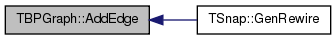int TBPGraph::AddEdge ( const TEdgeI & EdgeI )
inline

Adds an edge between EdgeI.GetLNId() and EdgeI.GetRNId() to the graph.

Definition at line 1119 of file graph.h.

1119 { return AddEdge(EdgeI.GetSrcNId(), EdgeI.GetDstNId()); }
int AddEdge(const int &LeftNId, const int &RightNId)
Adds an edge between a node LeftNId on the left and a node RightNId on the right side of the bipartit...
Definition: graph.cpp:705

Here is the call graph for this function:Here is the caller graph for this function: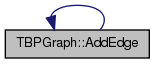int TBPGraph::AddNode ( int NId = `-1`, const bool & LeftNode = `true` )

Adds a node of ID NId to the graph.

Definition at line 671 of file graph.cpp.

References TStr::Fmt(), IAssertR, TMath::Mx(), and TNEGraph::MxNId.

Referenced by TSnap::GenRewire().

671  {
672  if (NId == -1) { NId = MxNId; MxNId++; }
673  else if (IsLNode(NId)) { IAssertR(LeftNode, TStr::Fmt("Node with id %s already exists on the 'left'.", NId)); return NId; }
674  else if (IsRNode(NId)) { IAssertR(! LeftNode, TStr::Fmt("Node with id %s already exists on the 'right'.", NId)); return NId; }
675  else { MxNId = TMath::Mx(NId+1, MxNId()); }
676  if (LeftNode) { LeftH.AddDat(NId, TNode(NId)); }
677  else { RightH.AddDat(NId, TNode(NId)); }
678  return NId;
679 }
#define IAssertR(Cond, Reason)
Definition: bd.h:265
static const T & Mx(const T &LVal, const T &RVal)
Definition: xmath.h:32
bool IsLNode(const int &NId) const
Tests whether ID NId is a 'left' side node.
Definition: graph.h:1092
TInt MxNId
Definition: graph.h:1046
static TStr Fmt(const char *FmtStr,...)
Definition: dt.cpp:1599
bool IsRNode(const int &NId) const
Tests whether ID NId is a 'right' side node.
Definition: graph.h:1094
THash< TInt, TNode > RightH
Definition: graph.h:1048
THash< TInt, TNode > LeftH
Definition: graph.h:1047
Definition: hash.h:238

Here is the call graph for this function: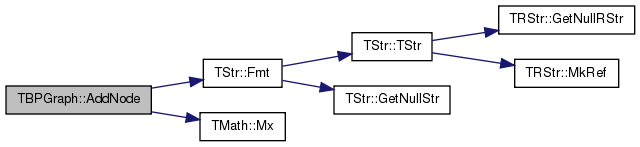Here is the caller graph for this function: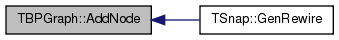int TBPGraph::AddNode ( const TNodeI & NodeI )
inline

Adds a node of ID NodeI.GetId() to the graph.

Definition at line 1083 of file graph.h.

1083 { return AddNode(NodeI.GetId(), NodeI.IsLeft()); }
int AddNode(int NId=-1, const bool &LeftNode=true)
Adds a node of ID NId to the graph.
Definition: graph.cpp:671

Here is the call graph for this function:Here is the caller graph for this function: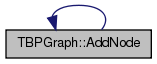TEdgeI TBPGraph::BegEI ( ) const
inline

Returns an iterator referring to the first edge in the graph.

Definition at line 1126 of file graph.h.

1126 { TNodeI NI=BegLNI(); while (NI<EndLNI() && (NI.GetOutDeg()==0 || NI.GetId()>NI.GetOutNId(0))) { NI++; } return TEdgeI(NI, EndLNI()); }
TNodeI BegLNI() const
Returns an iterator referring to the first 'left' node in the graph.
Definition: graph.h:1105
TNodeI EndLNI() const
Returns an iterator referring to the past-the-end 'left' node in the graph.
Definition: graph.h:1107

Here is the call graph for this function:TNodeI TBPGraph::BegLNI ( ) const
inline

Returns an iterator referring to the first 'left' node in the graph.

Definition at line 1105 of file graph.h.

Referenced by BegEI().

1105 { return TNodeI(LeftH.BegI(), RightH.EndI()); }
TIter BegI() const
Definition: hash.h:213
TIter EndI() const
Definition: hash.h:218
THash< TInt, TNode > RightH
Definition: graph.h:1048
THash< TInt, TNode > LeftH
Definition: graph.h:1047

Here is the call graph for this function:Here is the caller graph for this function: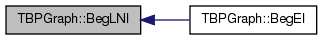TNodeI TBPGraph::BegNI ( ) const
inline

Returns an iterator referring to the first node in the graph.

Definition at line 1099 of file graph.h.

References THash< TKey, TDat, THashFunc >::BegI().

1099 { return TNodeI(LeftH.BegI(), RightH.BegI()); }
TIter BegI() const
Definition: hash.h:213
THash< TInt, TNode > RightH
Definition: graph.h:1048
THash< TInt, TNode > LeftH
Definition: graph.h:1047

Here is the call graph for this function: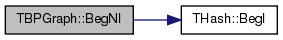TNodeI TBPGraph::BegRNI ( ) const
inline

Returns an iterator referring to the first 'right' node in the graph.

Definition at line 1109 of file graph.h.

1109 { return TNodeI(LeftH.EndI(), RightH.BegI()); }
TIter BegI() const
Definition: hash.h:213
TIter EndI() const
Definition: hash.h:218
THash< TInt, TNode > RightH
Definition: graph.h:1048
THash< TInt, TNode > LeftH
Definition: graph.h:1047

Here is the call graph for this function:void TBPGraph::Clr ( )
inline

Deletes all nodes and edges from the bipartite graph.

Definition at line 1153 of file graph.h.

References THash< TKey, TDat, THashFunc >::Clr().

1153 { MxNId=0; LeftH.Clr(); RightH.Clr(); }
TInt MxNId
Definition: graph.h:1046
THash< TInt, TNode > RightH
Definition: graph.h:1048
void Clr(const bool &DoDel=true, const int &NoDelLim=-1, const bool &ResetDat=true)
Definition: hash.h:361
THash< TInt, TNode > LeftH
Definition: graph.h:1047

Here is the call graph for this function: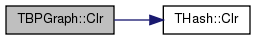void TBPGraph::Defrag ( const bool & OnlyNodeLinks = `false` )

Defragments the biparite graph.

Definition at line 794 of file graph.cpp.

794  {
795  for (int n = LeftH.FFirstKeyId(); LeftH.FNextKeyId(n); ) {
796  LeftH[n].NIdV.Pack(); }
797  for (int n = RightH.FFirstKeyId(); RightH.FNextKeyId(n); ) {
798  RightH[n].NIdV.Pack(); }
799  if (! OnlyNodeLinks && ! LeftH.IsKeyIdEqKeyN()) { LeftH.Defrag(); }
800  if (! OnlyNodeLinks && ! RightH.IsKeyIdEqKeyN()) { RightH.Defrag(); }
801 }
bool IsKeyIdEqKeyN() const
Definition: hash.h:233
void Defrag()
Definition: hash.h:555
bool FNextKeyId(int &KeyId) const
Definition: hash.h:478
int FFirstKeyId() const
Definition: hash.h:278
THash< TInt, TNode > RightH
Definition: graph.h:1048
THash< TInt, TNode > LeftH
Definition: graph.h:1047
void Pack()
Definition: hash.h:289
 void TBPGraph::DelEdge ( const int & LeftNId, const int & RightNId )

Deletes an edge between a node LeftNId on the left and a node RightNId on the right side of the bipartite graph.

Definition at line 719 of file graph.cpp.

719  {
720  const bool IsLL = IsLNode(LeftNId), IsLR = IsRNode(LeftNId);
721  const bool IsRL = IsLNode(RightNId), IsRR = IsRNode(RightNId);
722  IAssertR((IsLL||IsLR)&&(IsRL||IsRR), TStr::Fmt("%d or %d is not a node.", LeftNId, RightNId).CStr());
723  IAssertR(LeftNId!=RightNId, "No self-edges are allowed.");
724  IAssertR((IsLL&&!IsLR&&!IsRL&&IsRR)||(!IsLL&&IsLR&&IsRL&&!IsRR), "One node should be on the 'left' and the other on the 'right'.");
725  const int LNId = IsLL ? LeftNId : RightNId; // the real left node
726  const int RNId = IsLL ? RightNId : LeftNId; // the real right node
727  { TIntV& NIdV = LeftH.GetDat(LNId).NIdV;
728  const int n = NIdV.SearchBin(RNId);
729  if (n != -1) { NIdV.Del(n); } }
730  { TIntV& NIdV = RightH.GetDat(RNId).NIdV;
731  const int n = NIdV.SearchBin(LNId);
732  if (n != -1) { NIdV.Del(n); } }
733 }
#define IAssertR(Cond, Reason)
Definition: bd.h:265
void Del(const TSizeTy &ValN)
Removes the element at position ValN.
Definition: ds.h:1189
bool IsLNode(const int &NId) const
Tests whether ID NId is a 'left' side node.
Definition: graph.h:1092
const TDat & GetDat(const TKey &Key) const
Definition: hash.h:262
TIntV NIdV
Definition: graph.h:945
TSizeTy SearchBin(const TVal &Val) const
Returns the position of an element with value Val.
Definition: ds.h:1519
static TStr Fmt(const char *FmtStr,...)
Definition: dt.cpp:1599
bool IsRNode(const int &NId) const
Tests whether ID NId is a 'right' side node.
Definition: graph.h:1094
THash< TInt, TNode > RightH
Definition: graph.h:1048
THash< TInt, TNode > LeftH
Definition: graph.h:1047

Here is the call graph for this function: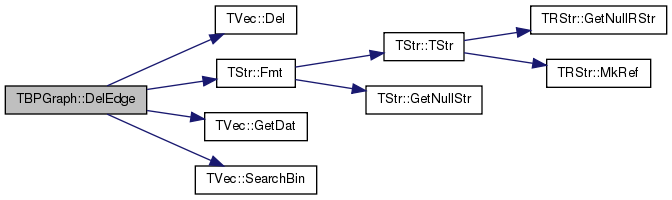void TBPGraph::DelNode ( const int & NId )

Deletes node of ID NId from the graph.

Definition at line 682 of file graph.cpp.

682  {
683  AssertR(IsNode(NId), TStr::Fmt("NodeId %d does not exist", NId));
684  THash<TInt, TNode>& SrcH = IsLNode(NId) ? LeftH : RightH;
685  THash<TInt, TNode>& DstH = IsLNode(NId) ? RightH : LeftH;
686  { TNode& Node = SrcH.GetDat(NId);
687  for (int e = 0; e < Node.GetOutDeg(); e++) {
688  const int nbr = Node.GetOutNId(e);
689  IAssertR(nbr != NId, "Bipartite graph has a loop!");
690  TNode& N = DstH.GetDat(nbr);
691  const int n = N.NIdV.SearchBin(NId);
692  IAssert(n!= -1);
693  N.NIdV.Del(n);
694  } }
695  SrcH.DelKey(NId);
696 }
#define IAssert(Cond)
Definition: bd.h:262
#define IAssertR(Cond, Reason)
Definition: bd.h:265
bool IsNode(const int &NId) const
Tests whether ID NId is a node.
Definition: graph.h:1090
bool IsLNode(const int &NId) const
Tests whether ID NId is a 'left' side node.
Definition: graph.h:1092
const TDat & GetDat(const TKey &Key) const
Definition: hash.h:262
int GetOutNId(const int &NodeN) const
Definition: graph.h:958
void DelKey(const TKey &Key)
Definition: hash.h:404
TIntV NIdV
Definition: graph.h:945
TSizeTy SearchBin(const TVal &Val) const
Returns the position of an element with value Val.
Definition: ds.h:1519
static TStr Fmt(const char *FmtStr,...)
Definition: dt.cpp:1599
THash< TInt, TNode > RightH
Definition: graph.h:1048
THash< TInt, TNode > LeftH
Definition: graph.h:1047
#define AssertR(Cond, Reason)
Definition: bd.h:258

Here is the call graph for this function: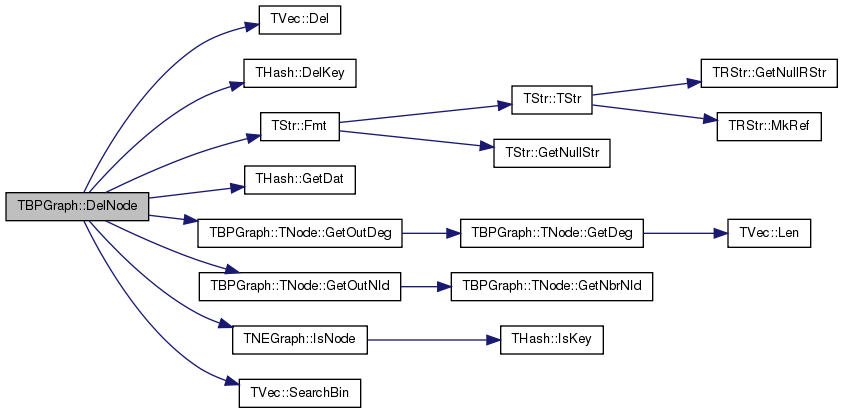void TBPGraph::DelNode ( const TNode & NodeI )
inline

Deletes node of ID NodeI.GetId() from the graph.

Definition at line 1088 of file graph.h.

References DelNode(), and TBPGraph::TNode::GetId().

Referenced by DelNode().

1088 { DelNode(NodeI.GetId()); }
void DelNode(const int &NId)
Deletes node of ID NId from the graph.
Definition: graph.cpp:682

Here is the call graph for this function:Here is the caller graph for this function: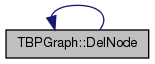void TBPGraph::Dump ( FILE * OutF = `stdout` ) const

Print the biparite graph in a human readable form to an output stream OutF.

Definition at line 845 of file graph.cpp.

845  {
846  const int NodePlaces = (int) ceil(log10((double) GetNodes()));
847  fprintf(OutF, "-------------------------------------------------\nBipartite Graph: nodes: %d+%d=%d, edges: %d\n", GetLNodes(), GetRNodes(), GetNodes(), GetEdges());
848  for (int N = LeftH.FFirstKeyId(); LeftH.FNextKeyId(N); ) {
849  const TNode& Node = LeftH[N];
850  fprintf(OutF, " %*d [%d] ", NodePlaces, Node.GetId(), Node.GetDeg());
851  for (int edge = 0; edge < Node.GetDeg(); edge++) {
852  fprintf(OutF, " %*d", NodePlaces, Node.GetNbrNId(edge)); }
853  fprintf(OutF, "\n");
854  }
855  fprintf(OutF, "\n");
856  /*// Also dump the 'right' side
857  fprintf(OutF, "\n");
858  for (int N = RightH.FFirstKeyId(); RightH.FNextKeyId(N); ) {
859  const TNode& Node = RightH[N];
860  fprintf(OutF, " %*d [%d] ", NodePlaces, Node.GetId(), Node.GetDeg());
861  for (int edge = 0; edge < Node.GetDeg(); edge++) {
862  fprintf(OutF, " %*d", NodePlaces, Node.GetNbrNId(edge)); }
863  fprintf(OutF, "\n");
864  }
865  fprintf(OutF, "\n"); //*/
866 }
int GetLNodes() const
Returns the number of nodes on the 'left' side of the biparite graph.
Definition: graph.h:1076
int GetEdges() const
Returns the number of edges in the graph.
Definition: graph.cpp:698
int GetNodes() const
Returns the total number of nodes in the graph.
Definition: graph.h:1074
int GetRNodes() const
Returns the number of nodes on the 'right' side of the biparite graph.
Definition: graph.h:1078
bool FNextKeyId(int &KeyId) const
Definition: hash.h:478
int FFirstKeyId() const
Definition: hash.h:278
THash< TInt, TNode > LeftH
Definition: graph.h:1047

Here is the call graph for this function: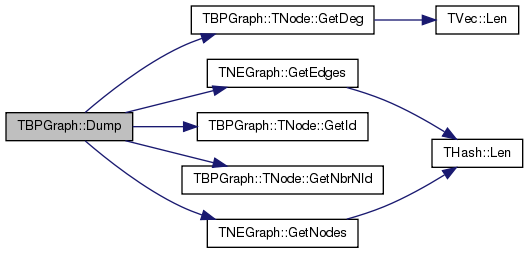bool TBPGraph::Empty ( ) const
inline

Tests whether the bipartite graph is empty (has zero nodes).

Definition at line 1151 of file graph.h.

References GetNodes().

1151 { return GetNodes()==0; }
int GetNodes() const
Returns the total number of nodes in the graph.
Definition: graph.h:1074

Here is the call graph for this function:TEdgeI TBPGraph::EndEI ( ) const
inline

Returns an iterator referring to the past-the-end edge in the graph.

Definition at line 1128 of file graph.h.

References EndNI().

1128 { return TEdgeI(EndNI(), EndNI()); }
TNodeI EndNI() const
Returns an iterator referring to the past-the-end node in the graph.
Definition: graph.h:1101

Here is the call graph for this function: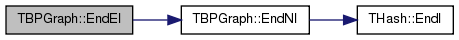TNodeI TBPGraph::EndLNI ( ) const
inline

Returns an iterator referring to the past-the-end 'left' node in the graph.

Definition at line 1107 of file graph.h.

References EndNI().

Referenced by BegEI().

1107 { return EndNI(); }
TNodeI EndNI() const
Returns an iterator referring to the past-the-end node in the graph.
Definition: graph.h:1101

Here is the call graph for this function: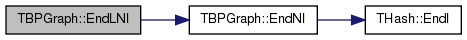Here is the caller graph for this function: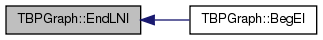TNodeI TBPGraph::EndNI ( ) const
inline

Returns an iterator referring to the past-the-end node in the graph.

Definition at line 1101 of file graph.h.

References THash< TKey, TDat, THashFunc >::EndI().

Referenced by EndEI(), EndLNI(), and EndRNI().

1101 { return TNodeI(LeftH.EndI(), RightH.EndI()); }
TIter EndI() const
Definition: hash.h:218
THash< TInt, TNode > RightH
Definition: graph.h:1048
THash< TInt, TNode > LeftH
Definition: graph.h:1047

Here is the call graph for this function: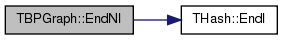Here is the caller graph for this function: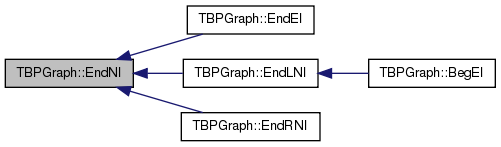TNodeI TBPGraph::EndRNI ( ) const
inline

Returns an iterator referring to the past-the-end 'right' node in the graph.

Definition at line 1111 of file graph.h.

References EndNI().

1111 { return EndNI(); }
TNodeI EndNI() const
Returns an iterator referring to the past-the-end node in the graph.
Definition: graph.h:1101

Here is the call graph for this function: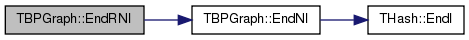int TBPGraph::GetEdges ( ) const

Returns the number of edges in the graph.

Definition at line 698 of file graph.cpp.

698  {
699  int Edges = 0;
700  for (int N=LeftH.FFirstKeyId(); LeftH.FNextKeyId(N); ) {
701  Edges += LeftH[N].GetDeg(); }
702  return Edges;
703 }
bool FNextKeyId(int &KeyId) const
Definition: hash.h:478
int FFirstKeyId() const
Definition: hash.h:278
THash< TInt, TNode > LeftH
Definition: graph.h:1047
 TEdgeI TBPGraph::GetEI ( const int & EId ) const

Not supported/implemented!

 TBPGraph::TEdgeI TBPGraph::GetEI ( const int & LeftNId, const int & RightNId ) const

Returns an iterator referring to edge (LeftNId, RightNId) in the graph.

Definition at line 740 of file graph.cpp.

740  {
741  const bool IsLL = IsLNode(LeftNId), IsLR = IsRNode(LeftNId);
742  const bool IsRL = IsLNode(RightNId), IsRR = IsRNode(RightNId);
743  IAssertR((IsLL||IsLR)&&(IsRL||IsRR), TStr::Fmt("%d or %d is not a node.", LeftNId, RightNId).CStr());
744  IAssertR(LeftNId!=RightNId, "No self-edges are allowed.");
745  IAssertR((IsLL&&!IsLR&&!IsRL&&IsRR)||(!IsLL&&IsLR&&IsRL&&!IsRR), "One node should be on the 'left' and the other on the 'right'.");
746  const int LNId = IsLL ? LeftNId : RightNId; // the real left node
747  const int RNId = IsLL ? RightNId : LeftNId; // the real right node
748  const TNodeI SrcNI = GetNI(LNId);
749  const int NodeN = SrcNI.LeftHI.GetDat().NIdV.SearchBin(RNId);
750  IAssertR(NodeN != -1, "Right edge endpoint does not exists!");
751  return TEdgeI(SrcNI, EndNI(), NodeN);
752 }
#define IAssertR(Cond, Reason)
Definition: bd.h:265
TNodeI GetNI(const int &NId) const
Returns an iterator referring to the node of ID NId in the graph.
Definition: graph.h:1103
bool IsLNode(const int &NId) const
Tests whether ID NId is a 'left' side node.
Definition: graph.h:1092
TNodeI EndNI() const
Returns an iterator referring to the past-the-end node in the graph.
Definition: graph.h:1101
static TStr Fmt(const char *FmtStr,...)
Definition: dt.cpp:1599
bool IsRNode(const int &NId) const
Tests whether ID NId is a 'right' side node.
Definition: graph.h:1094

Here is the call graph for this function: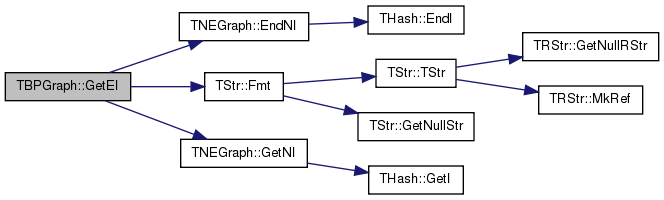void TBPGraph::GetLNIdV ( TIntV & NIdV ) const

Gets a vector IDs of all 'left' nodes in the bipartite graph.

Definition at line 778 of file graph.cpp.

References TVec< TVal, TSizeTy >::Add(), and TVec< TVal, TSizeTy >::Gen().

778  {
779  NIdV.Gen(GetLNodes(), 0);
780  for (int N=LeftH.FFirstKeyId(); LeftH.FNextKeyId(N); ) {
782 }
int GetLNodes() const
Returns the number of nodes on the 'left' side of the biparite graph.
Definition: graph.h:1076
bool FNextKeyId(int &KeyId) const
Definition: hash.h:478
int FFirstKeyId() const
Definition: hash.h:278
THash< TInt, TNode > LeftH
Definition: graph.h:1047
void Gen(const TSizeTy &_Vals)
Constructs a vector (an array) of _Vals elements.
Definition: ds.h:523
Adds a new element at the end of the vector, after its current last element.
Definition: ds.h:602
const TKey & GetKey(const int &KeyId) const
Definition: hash.h:252

Here is the call graph for this function:int TBPGraph::GetLNodes ( ) const
inline

Returns the number of nodes on the 'left' side of the biparite graph.

Definition at line 1076 of file graph.h.

References THash< TKey, TDat, THashFunc >::Len().

Referenced by GetNodes().

1076 { return LeftH.Len(); }
THash< TInt, TNode > LeftH
Definition: graph.h:1047
int Len() const
Definition: hash.h:228

Here is the call graph for this function: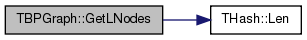Here is the caller graph for this function: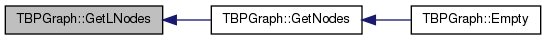int TBPGraph::GetMxNId ( ) const
inline

Returns an ID that is larger than any node ID in the graph.

Definition at line 1096 of file graph.h.

References MxNId.

1096 { return MxNId; }
TInt MxNId
Definition: graph.h:1046
 TNodeI TBPGraph::GetNI ( const int & NId ) const
inline

Returns an iterator referring to the node of ID NId in the graph.

Definition at line 1103 of file graph.h.

Referenced by GetRndNI().

1103 { return IsLNode(NId) ? TNodeI(LeftH.GetI(NId), RightH.EndI()) : TNodeI(LeftH.EndI(), RightH.GetI(NId)); }
bool IsLNode(const int &NId) const
Tests whether ID NId is a 'left' side node.
Definition: graph.h:1092
TIter EndI() const
Definition: hash.h:218
THash< TInt, TNode > RightH
Definition: graph.h:1048
THash< TInt, TNode > LeftH
Definition: graph.h:1047
TIter GetI(const TKey &Key) const
Definition: hash.h:220

Here is the call graph for this function: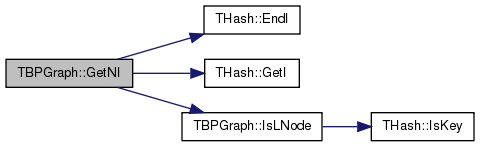Here is the caller graph for this function: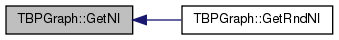void TBPGraph::GetNIdV ( TIntV & NIdV ) const

Gets a vector IDs of all nodes in the bipartite graph.

Definition at line 770 of file graph.cpp.

770  {
771  NIdV.Gen(GetNodes(), 0);
772  for (int N=LeftH.FFirstKeyId(); LeftH.FNextKeyId(N); ) {
774  for (int N=RightH.FFirstKeyId(); RightH.FNextKeyId(N); ) {
776 }
int GetNodes() const
Returns the total number of nodes in the graph.
Definition: graph.h:1074
bool FNextKeyId(int &KeyId) const
Definition: hash.h:478
int FFirstKeyId() const
Definition: hash.h:278
THash< TInt, TNode > RightH
Definition: graph.h:1048
THash< TInt, TNode > LeftH
Definition: graph.h:1047
void Gen(const TSizeTy &_Vals)
Constructs a vector (an array) of _Vals elements.
Definition: ds.h:523
Adds a new element at the end of the vector, after its current last element.
Definition: ds.h:602
const TKey & GetKey(const int &KeyId) const
Definition: hash.h:252

Here is the call graph for this function: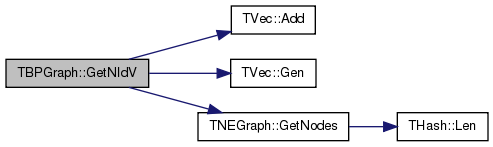int TBPGraph::GetNodes ( ) const
inline

Returns the total number of nodes in the graph.

Definition at line 1074 of file graph.h.

References GetLNodes(), and GetRNodes().

Referenced by Empty().

1074 { return GetLNodes() + GetRNodes(); }
int GetLNodes() const
Returns the number of nodes on the 'left' side of the biparite graph.
Definition: graph.h:1076
int GetRNodes() const
Returns the number of nodes on the 'right' side of the biparite graph.
Definition: graph.h:1078

Here is the call graph for this function:Here is the caller graph for this function: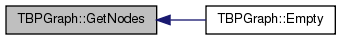int TBPGraph::GetRndLNId ( TRnd & Rnd = `TInt::Rnd` )

Returns an ID of a random 'left' node in the graph.

Definition at line 762 of file graph.cpp.

762  {
763  return LeftH.GetKey(LeftH.GetRndKeyId(Rnd, 0.8));
764 }
THash< TInt, TNode > LeftH
Definition: graph.h:1047
int GetRndKeyId(TRnd &Rnd) const
Get an index of a random element. If the hash table has many deleted keys, this may take a long time...
Definition: hash.h:444
const TKey & GetKey(const int &KeyId) const
Definition: hash.h:252
 TNodeI TBPGraph::GetRndNI ( TRnd & Rnd = `TInt::Rnd` )
inline

Returns an interator referring to a random node in the graph.

Definition at line 1142 of file graph.h.

References GetNI(), and GetRndNId().

1142 { return GetNI(GetRndNId(Rnd)); }
TNodeI GetNI(const int &NId) const
Returns an iterator referring to the node of ID NId in the graph.
Definition: graph.h:1103
int GetRndNId(TRnd &Rnd=TInt::Rnd)
Returns an ID of a random node in the graph.
Definition: graph.cpp:754

Here is the call graph for this function: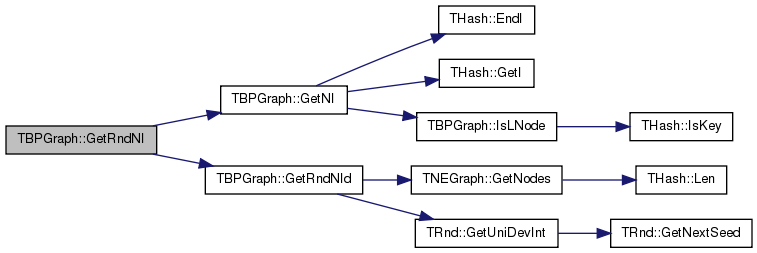int TBPGraph::GetRndNId ( TRnd & Rnd = `TInt::Rnd` )

Returns an ID of a random node in the graph.

Definition at line 754 of file graph.cpp.

References TNEGraph::GetNodes(), and TRnd::GetUniDevInt().

Referenced by GetRndNI().

754  {
755  const int NNodes = GetNodes();
756  if (Rnd.GetUniDevInt(NNodes) < GetLNodes()) {
757  return GetRndLNId(Rnd); }
758  else {
759  return GetRndRNId(Rnd); }
760 }
int GetRndLNId(TRnd &Rnd=TInt::Rnd)
Returns an ID of a random 'left' node in the graph.
Definition: graph.cpp:762
int GetLNodes() const
Returns the number of nodes on the 'left' side of the biparite graph.
Definition: graph.h:1076
int GetNodes() const
Returns the total number of nodes in the graph.
Definition: graph.h:1074
int GetRndRNId(TRnd &Rnd=TInt::Rnd)
Returns an ID of a random 'right' node in the graph.
Definition: graph.cpp:766
int GetUniDevInt(const int &Range=0)
Definition: dt.cpp:39

Here is the call graph for this function:Here is the caller graph for this function: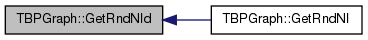int TBPGraph::GetRndRNId ( TRnd & Rnd = `TInt::Rnd` )

Returns an ID of a random 'right' node in the graph.

Definition at line 766 of file graph.cpp.

766  {
767  return RightH.GetKey(RightH.GetRndKeyId(Rnd, 0.8));
768 }
THash< TInt, TNode > RightH
Definition: graph.h:1048
int GetRndKeyId(TRnd &Rnd) const
Get an index of a random element. If the hash table has many deleted keys, this may take a long time...
Definition: hash.h:444
const TKey & GetKey(const int &KeyId) const
Definition: hash.h:252
 void TBPGraph::GetRNIdV ( TIntV & NIdV ) const

Gets a vector IDs of all 'right' nodes in the bipartite graph.

Definition at line 784 of file graph.cpp.

References TVec< TVal, TSizeTy >::Add(), and TVec< TVal, TSizeTy >::Gen().

784  {
785  NIdV.Gen(GetRNodes(), 0);
786  for (int N=RightH.FFirstKeyId(); RightH.FNextKeyId(N); ) {
788 }
int GetRNodes() const
Returns the number of nodes on the 'right' side of the biparite graph.
Definition: graph.h:1078
bool FNextKeyId(int &KeyId) const
Definition: hash.h:478
int FFirstKeyId() const
Definition: hash.h:278
THash< TInt, TNode > RightH
Definition: graph.h:1048
void Gen(const TSizeTy &_Vals)
Constructs a vector (an array) of _Vals elements.
Definition: ds.h:523
Adds a new element at the end of the vector, after its current last element.
Definition: ds.h:602
const TKey & GetKey(const int &KeyId) const
Definition: hash.h:252

Here is the call graph for this function:int TBPGraph::GetRNodes ( ) const
inline

Returns the number of nodes on the 'right' side of the biparite graph.

Definition at line 1078 of file graph.h.

References THash< TKey, TDat, THashFunc >::Len().

Referenced by GetNodes().

1078 { return RightH.Len(); }
THash< TInt, TNode > RightH
Definition: graph.h:1048
int Len() const
Definition: hash.h:228

Here is the call graph for this function: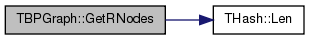Here is the caller graph for this function: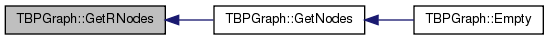PBPGraph TBPGraph::GetSmallGraph ( )
static

Returns a small graph on 2 'left', 3 'right' nodes and 4 edges.

Definition at line 868 of file graph.cpp.

References New().

868  {
869  PBPGraph BP = TBPGraph::New();
880  return BP;
881 }
static PBPGraph New()
Static constructor that returns a pointer to the graph. Call: PBPGraph BPGraph = TBPGraph::New();.
Definition: graph.h:1062
Definition: bd.h:196

Here is the call graph for this function: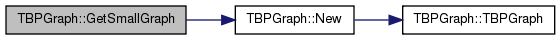bool TBPGraph::HasFlag ( const TGraphFlag & Flag ) const

Allows for run-time checking the type of the graph (see the TGraphFlag for flags).

 bool TBPGraph::IsEdge ( const int & LeftNId, const int & RightNId ) const

Tests whether an edge between node IDs LeftNId and RightNId exists in the graph.

Definition at line 735 of file graph.cpp.

References TNEGraph::IsNode().

735  {
736  if (! IsNode(LeftNId) || ! IsNode(RightNId)) { return false; }
737  return IsLNode(LeftNId) ? LeftH.GetDat(LeftNId).IsOutNId(RightNId) : RightH.GetDat(LeftNId).IsOutNId(RightNId);
738 }
bool IsNode(const int &NId) const
Tests whether ID NId is a node.
Definition: graph.h:1090
bool IsLNode(const int &NId) const
Tests whether ID NId is a 'left' side node.
Definition: graph.h:1092
const TDat & GetDat(const TKey &Key) const
Definition: hash.h:262
bool IsOutNId(const int &NId) const
Definition: graph.h:962
THash< TInt, TNode > RightH
Definition: graph.h:1048
THash< TInt, TNode > LeftH
Definition: graph.h:1047

Here is the call graph for this function: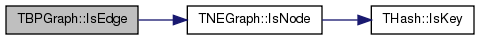bool TBPGraph::IsLNode ( const int & NId ) const
inline

Tests whether ID NId is a 'left' side node.

Definition at line 1092 of file graph.h.

References THash< TKey, TDat, THashFunc >::IsKey().

Referenced by GetNI(), and IsNode().

1092 { return LeftH.IsKey(NId); }
THash< TInt, TNode > LeftH
Definition: graph.h:1047
bool IsKey(const TKey &Key) const
Definition: hash.h:258

Here is the call graph for this function: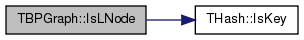Here is the caller graph for this function:bool TBPGraph::IsNode ( const int & NId ) const
inline

Tests whether ID NId is a node.

Definition at line 1090 of file graph.h.

References IsLNode(), and IsRNode().

1090 { return IsLNode(NId) || IsRNode(NId); }
bool IsLNode(const int &NId) const
Tests whether ID NId is a 'left' side node.
Definition: graph.h:1092
bool IsRNode(const int &NId) const
Tests whether ID NId is a 'right' side node.
Definition: graph.h:1094

Here is the call graph for this function:bool TBPGraph::IsOk ( const bool & ThrowExcept = `true` ) const

Checks the bipartite graph data structure for internal consistency.

Definition at line 803 of file graph.cpp.

References TStr::CStr(), EAssertR, ErrNotify(), and TStr::Fmt().

803  {
804  bool RetVal = false;
805  // edge lists are sorted
806  for (int n = LeftH.FFirstKeyId(); LeftH.FNextKeyId(n); ) {
807  if (! LeftH[n].NIdV.IsSorted()) {
808  const TStr Msg = TStr::Fmt("Neighbor list of node %d is not sorted.", LeftH[n].GetId());
809  if (ThrowExcept) { EAssertR(false, Msg); } else { ErrNotify(Msg.CStr()); } RetVal=false; }
810  }
811  for (int n = RightH.FFirstKeyId(); RightH.FNextKeyId(n); ) {
812  if (! RightH[n].NIdV.IsSorted()) {
813  const TStr Msg = TStr::Fmt("Neighbor list of node %d is not sorted.", RightH[n].GetId());
814  if (ThrowExcept) { EAssertR(false, Msg); } else { ErrNotify(Msg.CStr()); } RetVal=false; }
815  }
816  // nodes only appear on one side
817  for (int n = LeftH.FFirstKeyId(); LeftH.FNextKeyId(n); ) {
818  if (RightH.IsKey(LeftH[n].GetId())) {
819  const TStr Msg = TStr::Fmt("'Left' node %d also appears on the 'right'.", LeftH[n].GetId());
820  if (ThrowExcept) { EAssertR(false, Msg); } else { ErrNotify(Msg.CStr()); } RetVal=false; }
821  }
822  for (int n = RightH.FFirstKeyId(); RightH.FNextKeyId(n); ) {
823  if (LeftH.IsKey(RightH[n].GetId())) {
824  const TStr Msg = TStr::Fmt("'Right' node %d also appears on the 'left'.", RightH[n].GetId());
825  if (ThrowExcept) { EAssertR(false, Msg); } else { ErrNotify(Msg.CStr()); } RetVal=false; }
826  }
827  // edges only point from left to right and right to left
828  for (int n = LeftH.FFirstKeyId(); LeftH.FNextKeyId(n); ) {
829  for (int e = 0; e < LeftH[n].NIdV.Len(); e++) {
830  if (! RightH.IsKey(LeftH[n].NIdV[e]) || ! RightH.GetDat(LeftH[n].NIdV[e]).NIdV.IsIn(LeftH[n].GetId())) {
831  const TStr Msg = TStr::Fmt("'Left' node %d does not point to the 'right' node %d.", LeftH[n].GetId(), LeftH[n].NIdV[e]());
832  if (ThrowExcept) { EAssertR(false, Msg); } else { ErrNotify(Msg.CStr()); } RetVal=false; }
833  }
834  }
835  for (int n = RightH.FFirstKeyId(); RightH.FNextKeyId(n); ) {
836  for (int e = 0; e < RightH[n].NIdV.Len(); e++) {
837  if (! LeftH.IsKey(RightH[n].NIdV[e]) || ! LeftH.GetDat(RightH[n].NIdV[e]).NIdV.IsIn(RightH[n].GetId())) {
838  const TStr Msg = TStr::Fmt("'Left' node %d does not point to the 'right' node %d.", RightH[n].GetId(), RightH[n].NIdV[e]());
839  if (ThrowExcept) { EAssertR(false, Msg); } else { ErrNotify(Msg.CStr()); } RetVal=false; }
840  }
841  }
842  return RetVal;
843 }
bool IsIn(const TVal &Val) const
Checks whether element Val is a member of the vector.
Definition: ds.h:828
const TDat & GetDat(const TKey &Key) const
Definition: hash.h:262
void ErrNotify(const char *NotifyCStr)
Definition: bd.h:74
bool FNextKeyId(int &KeyId) const
Definition: hash.h:478
int FFirstKeyId() const
Definition: hash.h:278
TIntV NIdV
Definition: graph.h:945
Definition: dt.h:412
static TStr Fmt(const char *FmtStr,...)
Definition: dt.cpp:1599
THash< TInt, TNode > RightH
Definition: graph.h:1048
#define EAssertR(Cond, MsgStr)
Definition: bd.h:283
THash< TInt, TNode > LeftH
Definition: graph.h:1047
char * CStr()
Definition: dt.h:479
bool IsKey(const TKey &Key) const
Definition: hash.h:258
int Len() const
Definition: hash.h:228

Here is the call graph for this function: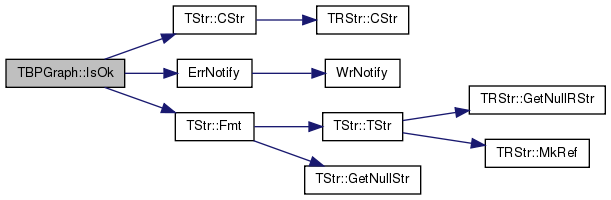bool TBPGraph::IsRNode ( const int & NId ) const
inline

Tests whether ID NId is a 'right' side node.

Definition at line 1094 of file graph.h.

References THash< TKey, TDat, THashFunc >::IsKey().

Referenced by IsNode().

1094 { return RightH.IsKey(NId); }
THash< TInt, TNode > RightH
Definition: graph.h:1048
bool IsKey(const TKey &Key) const
Definition: hash.h:258

Here is the call graph for this function: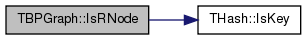Here is the caller graph for this function: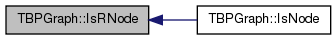static PBPGraph TBPGraph::Load ( TSIn & SIn )
inlinestatic

Static constructor that loads the graph from a stream SIn and returns a pointer to it.

Definition at line 1067 of file graph.h.

References TBPGraph().

1067 { return PBPGraph(new TBPGraph(SIn)); }
TBPGraph()
Definition: graph.h:1053
TPt< TBPGraph > PBPGraph
Pointer to a bipartitegraph graph (TBPGraph)
Definition: graph.h:11

Here is the call graph for this function: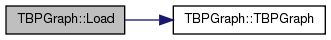static PBPGraph TBPGraph::New ( )
inlinestatic

Static constructor that returns a pointer to the graph. Call: PBPGraph BPGraph = TBPGraph::New();.

Definition at line 1062 of file graph.h.

References TBPGraph().

Referenced by TSnap::GenRewire(), TSnap::GenRndBipart(), and GetSmallGraph().

1062 { return new TBPGraph(); }
TBPGraph()
Definition: graph.h:1053

Here is the call graph for this function: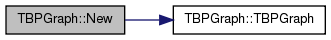Here is the caller graph for this function:static PBPGraph TBPGraph::New ( const int & Nodes, const int & Edges )
inlinestatic

Static constructor that returns a pointer to the graph and reserves enough memory for Nodes nodes and Edges edges.

Definition at line 1065 of file graph.h.

References TBPGraph().

1065 { return new TBPGraph(Nodes, Edges); }
TBPGraph()
Definition: graph.h:1053

Here is the call graph for this function:TBPGraph& TBPGraph::operator= ( const TBPGraph & BPGraph )
inline

Definition at line 1070 of file graph.h.

References LeftH, MxNId, and RightH.

1070  {
1071  if (this!=&BPGraph) { MxNId=BPGraph.MxNId; LeftH=BPGraph.LeftH; RightH=BPGraph.RightH; } return *this; }
TInt MxNId
Definition: graph.h:1046
THash< TInt, TNode > RightH
Definition: graph.h:1048
THash< TInt, TNode > LeftH
Definition: graph.h:1047
 void TBPGraph::Reserve ( const int & Nodes, const int & Edges )

Reserves memory for a biparite graph of Nodes nodes and Edges edges.

Definition at line 790 of file graph.cpp.

Referenced by TSnap::GenRewire().

790  {
791  if (Nodes>0) { LeftH.Gen(Nodes/2); RightH.Gen(Nodes/2); }
792 }
void Gen(const int &ExpectVals)
Definition: hash.h:222
THash< TInt, TNode > RightH
Definition: graph.h:1048
THash< TInt, TNode > LeftH
Definition: graph.h:1047

Here is the caller graph for this function: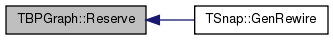void TBPGraph::Save ( TSOut & SOut ) const
inline

Saves the graph to a (binary) stream SOut.

Definition at line 1060 of file graph.h.

References THash< TKey, TDat, THashFunc >::Save(), and TInt::Save().

1060 { MxNId.Save(SOut); LeftH.Save(SOut); RightH.Save(SOut); }
void Save(TSOut &SOut) const
Definition: dt.h:1153
void Save(TSOut &SOut) const
Definition: hash.h:183
TInt MxNId
Definition: graph.h:1046
THash< TInt, TNode > RightH
Definition: graph.h:1048
THash< TInt, TNode > LeftH
Definition: graph.h:1047

Here is the call graph for this function:## Friends And Related Function Documentation

 friend class TPt< TBPGraph >
friend

Definition at line 1168 of file graph.h.

## Member Data Documentation

 TCRef TBPGraph::CRef
private

Definition at line 1045 of file graph.h.

 THash TBPGraph::LeftH
private

Definition at line 1047 of file graph.h.

Referenced by operator=().

 TInt TBPGraph::MxNId
private

Definition at line 1046 of file graph.h.

Referenced by GetMxNId(), and operator=().

 THash TBPGraph::RightH
private

Definition at line 1048 of file graph.h.

Referenced by operator=().

The documentation for this class was generated from the following files: